Home    |    Teacher    |    Parents    |    Glossary    |    About UsThe zero and the counting numbers (1,2,3,...) make up the set of whole numbers. But not every number is a whole number. Our decimal system lets us write numbers of all types and sizes, using a clever symbol called the decimal point.

As you move right from the decimal point, each place value is divided by 10.

Move your mouse cursor over the decimal number below to see its parts.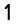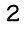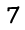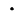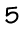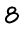Homework Help | Pre-Algebra | NumbersEmail this page to a friendSearch·  Place value·  Decimal numbers·  Estimating and     rounding·  Adding / subtracting     decimals·  Multiplying decimals·  Dividing decimals·  Percent·  Exponents·  Square roots·  Signed integers·  Adding and     subtracting integers·  Multiplying and     dividing integers·  Properties of integers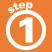First Glance In Depth Examples WorkoutDecimal numbers